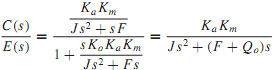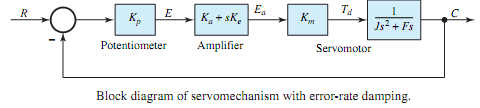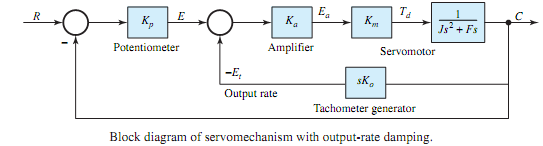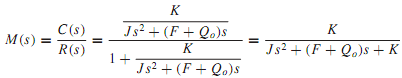## Illustrate output-rate control, Electrical Engineering

Assignment Help:

Q. Illustrate Output-rate control?

A system is said to have output-rate damping when the generation of the output quantity in some way ismade to depend upon the rate atwhich the controlled variable is varying.Output-rate control often involves the creation of an auxiliary loop, making the system multiloop. For the system of Figure, output-rate damping can be obtained by means of a tachometer generator, driven from the servomotor shaft. The complete block diagram of the servomechanism with output-rate damping is depicted in Figure, where Ko is the output-rate gain factor (V·s/rad) and the output-rate signal is given by Ko(dc/dt). By applying the feedback relationship of Equation to the minor (inner) loop, one getswhere Qo = KoKaKm is known as the loop output-rate gain factor. Figure may then be simplified. The closed-loop transfer function for the complete system is then given bywhere K = KpKaKm is known as the loop proportional gain factor, as stated earlier.

The manner in which output-rate damping effects in controlling the transient response are most easily demonstrated is by assuming a step input applied to the system. The output-rate signal appears in opposition to the proportional signal, thereby removing the tendency for an excessively oscillatory response.With error-rate damping and output-rate damping, the damping ratio can be seen to bewhere Q = Qe or Qo, in which a term is added to the numerator, while the natural frequency is unchanged (as given by ωn = √K/J ). The damping ratio can then be adjusted independently through Qe or Qo, while K can be used to meet accuracy requirements.

#### Why two rectifier is used in smps, Its not essential that a power plant use...

Its not essential that a power plant uses only two rectifiers, it depends on the load there at the site and the capacity of the rectifier or SMPS. Assume the load at the site is 12

#### How you can convert a galvanometer to a voltmeter, To convert a galvanomete...

To convert a galvanometer to a voltmeter, you should add a: a) High resistance in series b) High resistance in parallel c) Low resistance in series d) Low resis

#### Calculate the ratio v/i for an ideal diode, Q. Assuming the diode to obey I...

Q. Assuming the diode to obey I = IS (e V/0.026 -1), calculate the ratio V/I for an ideal diode with I S = 10 -13 A for the applied voltages of -2,-0.5, 0.3, 0.5, 0.7, 1.0, and

#### Help assignments, A three-phase transposed line is composed of one conducto...

A three-phase transposed line is composed of one conductor per phase with flat horizontal spacing of 11 m as shown in Figure 1(a). The conductors have a diameter of 3.625 cm and a

#### Fet operation, FET operation: Figure: I-V characteristics and o...

FET operation: Figure: I-V characteristics and output plot of a JFET n-channel transistor. The FET manages the flow of electrons (or electron holes) from the sourc

#### PLC Programming, FIG. 2 Car Park Simulation Consider the above diagram, FI...

FIG. 2 Car Park Simulation Consider the above diagram, FIGURE 2, of the car park. The object of this assignment is to write a program to operate the barriers to allow the cars in

#### Sketch the output waveform for the synchronous counter, Q. Consider a serie...

Q. Consider a series-carry synchronous counter with T flip-flops shown in Figure in which the AND gates carry forward the transitions of the flip-flops, thereby improving the speed

#### Resultant of two simple harmonic motion, how two make harmonic motion

how two make harmonic motion

#### Linear time invariant, A LIT (linear time-invariant) system, impulse respon...

A LIT (linear time-invariant) system, impulse response h [ n ], is described by: (a) Show the block-diagram representation for this FIR filter (only use multiplier, adder

#### Network theorem, what is couple circuit ?

what is couple circuit ?